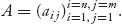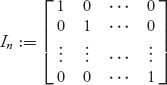Appendix A: The Essentials of Matrix Analysis

A matrix is a rectangular collection of numbers. If there are n rows and m columns, we write the matrix as An × m, and the numbers of the matrix are aij, where i gives the row number and j gives the column number. These are also called the dimensions of the matrix. We compactly writeIn rectangular formIf n = m, we say that the matrix is square. The square matrix in which there are all 1s along the diagonal and 0s everywhere else is called the identity matrix:Here are some facts about algebra with matrices:

1. We add two matrices that have the same dimensions, A + B, by adding the respective components A + B = (aij + bij), or2. A matrix may be multiplied by a scalar c by multiplying every element of A by c; that is, c A = (c aij) or3. We may multiply two matrices An×m and Bm × k only if the number of columns of A is exactly the same as the number of rows of B. You have to be careful because not only is A · BB · A; in general, it ...

Get Game Theory: An Introduction, 2nd Edition now with the O’Reilly learning platform.

O’Reilly members experience live online training, plus books, videos, and digital content from nearly 200 publishers.